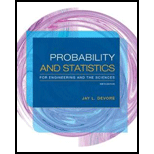# The simple Poisson process of Section 3.6 is characterized by a constant rate α at which events occur per unit time. A generalization of this is to suppose that the probability of exactly one event occurring in the interval [ t, t + Δ t ] is α ( t ) · Δ t + o (Δ t ). It can then be shown that the number of events occurring during an interval ( t 1 , t 2 ] has a Poisson distribution with parameter μ = ∫ t 2 t 1 α ( t ) d t The occurrence of events over time in this situation is called a nonhomogeneous Poisson process. The article ‘inference Based on Retrospective Ascertainment ” ( J. Amer. Stat. Assoc., 1989: 360-372). considers the intensity function α ( t ) = e a + b t as appropriate for events involving transmission of HIV (the AIDS virus) via blood transfusions. Suppose that a = 2 and b = .6 (close to values suggested in the paper), with time in years. a. What is the expected number of events in the interval [0, 4]? In [2, 6]? b. What is the probability that at most 15 events occur in the interval [0, .9907]?### Probability and Statistics for Eng...

9th Edition
Jay L. Devore
Publisher: Cengage Learning
ISBN: 9781305251809### Probability and Statistics for Eng...

9th Edition
Jay L. Devore
Publisher: Cengage Learning
ISBN: 9781305251809

#### Solutions

Chapter
Section
Chapter 3, Problem 120SE
Textbook Problem

## Expert Solution

### Want to see the full answer?

Check out a sample textbook solution.See solution

### Want to see this answer and more?

Experts are waiting 24/7 to provide step-by-step solutions in as fast as 30 minutes!*

See Solution

*Response times vary by subject and question complexity. Median response time is 34 minutes and may be longer for new subjects.